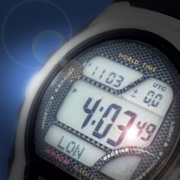< Back# Regular Expression to Find a Year

Written: 2012-04-10

## Introduction

Regular expressions are used heavily in programming languages such as Perl to search through text strings and locate patterns. If a regular expression is written poorly, it may either allow invalid values to be entered or will prohibit valid values to be entered.

A year can be entered as either a two digit or four digit number. Although since Y2K, it is much more common for years to be written with a full four digits, input received from a user should not assume that all four digits were entered.

Valid Years 95, 1900, 2012, 12, 0001 192, 2

## Regular Expression

A first attempt at a regular expression might be:

```if (\$str =~ /([0-9]{2,4})/) {
\$year = \$1;
}
```

But, this will match numbers within larger numbers. For example, given the string "8675309", this regular expression will match the year '8675'. Therefore, we can add the `\b` control sequence to ensure that we do not match numbers within larger words or numbers:

```if (\$str =~ /\b([0-9]{2,4})\b/) {
\$year = \$1;
}
```

The regular expression still has a small problem. The `[0-9]{2,4}` sequence looks for two, three or four consecutive digits, but we do not want the expression to match if it finds just three digits (e.g. 100), so we can change the expression one more time:

```if (\$str =~ /\b([0-9]{2}(?:[0-9]{2})?)\b/) {
\$year = \$1;
}
```

Now the regular expression correctly finds two digit or four digit numbers.

## Correcting Two-Digit Years

If a two-digit year is entered, it should be converted to a complete four-digit year. This is done by appending the current century to the value:

```if (length(\$year) == 2) {
\$year += 100 * int(\$current_year / 100);
```

However, if the current year is 2012 and the user enters '95', it is safe to assume that they intended 1995, not 2095. Because of this, after the current century has been appended to the year, we check to see if the year is within 50 years of the current year. If the year is not within 50 years, the century is adjusted accordingly:

```	if(\$year - \$current_year > 50) {
\$year -= 100;
} elsif (\$year - \$current_year < -50) {
\$year += 100;
}
}
```

If it is desired that only future years be allowed, then the code would instead need to check whether the year is less than the current year:

```	if(\$year < \$current_year) {
\$year += 100;
}
}
```

and if only past years are allowed, the code would instead need to check whether the year is greater than the current year:

```	if(\$year > \$current_year) {
\$year -= 100;
}
}
```

## Putting It Together

Combined together, the algorithm for identifying a year from a string, written in Perl, is:

```if (\$str =~ /\b([0-9]{2}(?:[0-9]{2})?)\b/) {
\$year = \$1;
if (length(\$year) == 2) {
\$year += 100 * int(\$current_year / 100);
if(\$year - \$current_year > 50) {
\$year -= 100;
} elsif (\$year - \$current_year < -50) {
\$year += 100;
}
}
}
```

Written in JavaScript, the algorithm is:

```var patt = /\b([0-9]{2}(?:[0-9]{2})?)\b/;
var match = patt.exec(str);
if (match) {
year = parseInt(match, 10);
if (match.length === 2) {
year += 100 * Math.floor(current_year / 100);
if(year - current_year > 50) {
year -= 100;
} elsif (year - current_year < -50) {
year += 100;
}
}
}
```